# 基于银企间多层金融网络的系统性风险传导机制及建模研究Systematic Risk Contagion Mechanism and Modeling Based on Multi-Layer Financial Network between Banks and Firms

• 全文下载: PDF(1367KB)    PP.350-364   DOI: 10.12677/FIN.2019.94043
• 下载量: 355  浏览量: 642   国家自然科学基金支持

Although the various relationships in the financial system have improved the convenience of fi-nancial services, they also make the risk contagion more complicated. This paper is devoted to the construction of a multi-layer financial network between banks and firms, including interbank lending, bank-firm lending and inter-firm guarantee relationships. Based on the balance sheet and off-balance-sheet business of banks and firms, we explain the relationship of rights and responsi-bilities between the various entities and clarify the risk components. Considering factors such as individual risk, credit risk and market liquidity risk, we describe the risk contagion mechanism from the perspective of layered conduction and step-by-step infection, and propose the contagion effect in the network structure. At the same time, based on the Debtrank algorithm, we construct a methodological approach to the individual and overall risk status in the risk conduction process.

1. 引言

2. 基于银企间多层金融网络的模型构建

2.1. 银企间网络模型的构成要素

${A}_{ji,\gamma }={\left\{{a}_{ji,\gamma }\right\}}_{N*N\left(M\right)}$ 来表示各节点之间的关系， $\gamma$ 表示不同层：银行间同业拆借层(第1层)、银企间借贷层

(第2层)、企业担保层(第3层)； ${a}_{ji,\gamma }$ 表示第 $\gamma$ 层上的第i个节点拥有第j个节点的债权金额，相当于i的风险敞口，按惯例方向是从节点j指向节点i；统一定义 ${a}_{ii,\gamma }=0$ 表示不存在自己对自己的债权。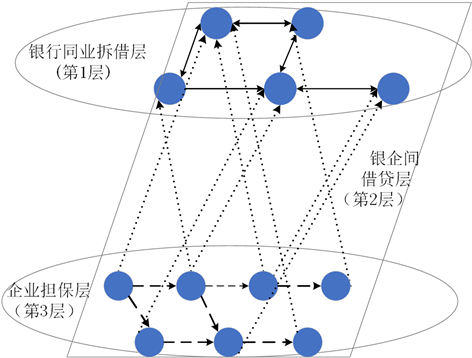Figure 1. Simplified network structure

2.2. 银行和企业两类主体的微观结构

${L}^{OF}$ 和权益 ${E}_{f}$ ；显然两张资产负债表中，企业的银企间负债与银行的银企间资产是相互对应的；权益 ${E}_{f}$ 作为资产缓冲器， ${E}_{f}\left(0\right)={\varphi }_{4}{V}_{f}\left(0\right)$ ；且 ${V}^{LF}\text{+}{V}^{OF}={L}^{BF}+{L}^{OF}+{E}_{f}$ 关系恒定；另外，由于担保业务属于表外业务，而企业对外提供担保实际上形成了或有负债，因而一旦企业被要求履行担保义务时，体现在资产负债表上为资产受损，这里假定流动性资产受损。Table 1. Simplified bank balance sheetTable 2. Simplified firm balance sheet

3. 基于银企间多层金融网络的风险传导机制

3.1. 简化的风险传导机制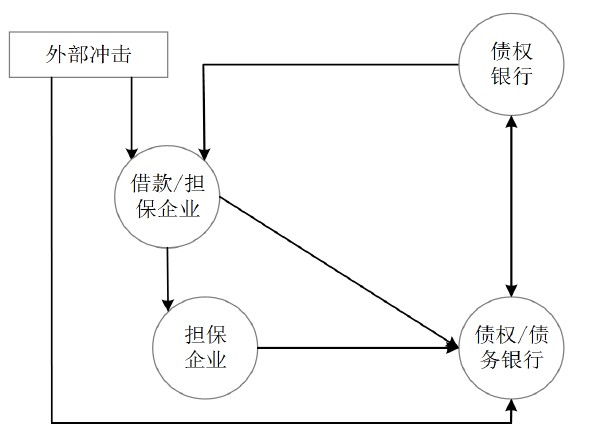Figure 2. Simplified risk conduction mechanism diagram

3.2. 银行同业拆借层的风险传导机制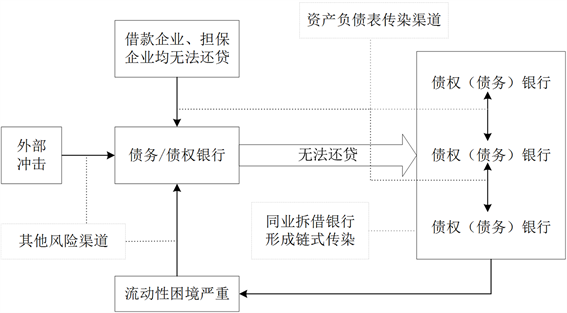Figure 3. Risk contagion mechanism of the interbank lending layer

3.3. 银企间借贷层的风险传导机制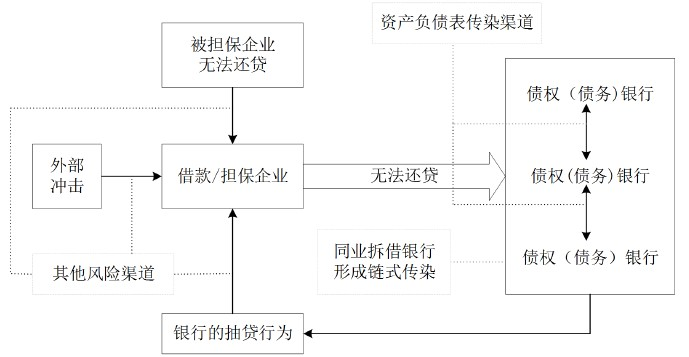Figure 4. Risk contagion mechanism of the lending layer between banks and firms

3.4. 企业担保层的风险传导机制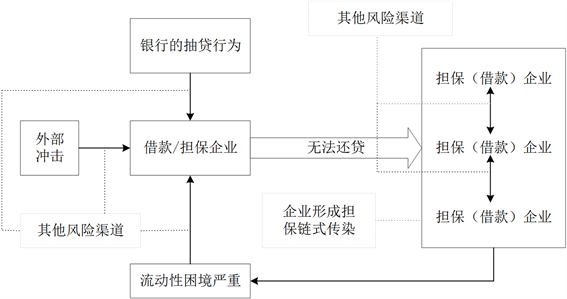Figure 5. Risk contagion mechanism of the firm guarantee layer

3.5. 分步骤的风险传导机制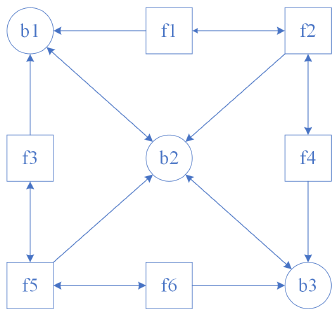(1)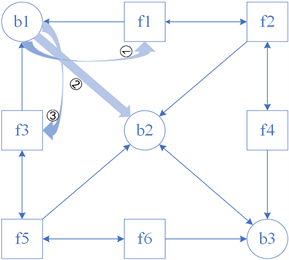(2)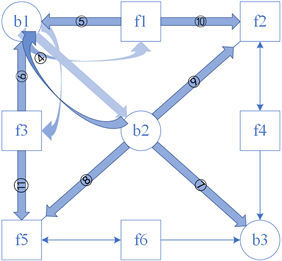(3)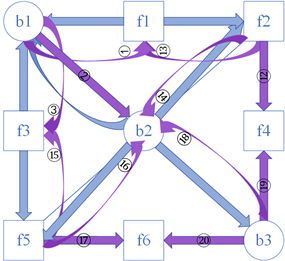(4)

Figure 6. Steps of the risk contagion mechanism

f5多次受到风险的传导。

4. 基于银企间多层金融网络的模型思路分析

4.1. 对风险状态的测度思路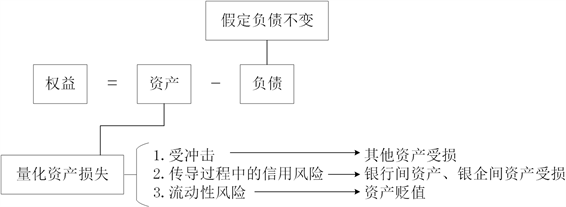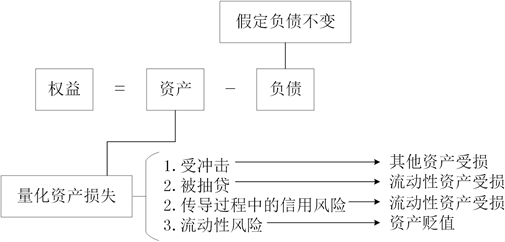4.2. 基于Debtrank算法的风险测度模型构建

${H}_{i}\left(t\right)=\mathrm{min}\left\{1,{H}_{i}\left(t-1\right)+\underset{{C}_{j}\left(t-1\right)=D}{\sum }{W}_{ji}{H}_{j}\left(t-1\right)\right\}$ (1)

${S}_{i}\left(t\right)=\left\{\begin{array}{l}D,{H}_{i}\left(t\right)>0;{S}_{i}\left(t-1\right)\ne I\\ I,{S}_{i}\left(t-1\right)=D\\ {S}_{i}\left(t-1\right),\text{otherwise}\end{array}$ (2)

Debtrank算法是一种受反馈中心性启发的新颖度量方法，能够量化在外部冲击下，特定节点产生财务困境并将风险扩散至整个网络的程度。Battiston等(2012)  将整个网络归一化，定义整个市场的总资

$L{O}_{f}=m{V}^{OF}\left(0\right)+\underset{{E}_{b}\left(t-1\right)\le 0}{\sum }{a}_{fb}\left(t\right)+{a}_{kf,3}\left(t\right)+\frac{1-q}{q}{V}^{OF}\left(t\right)$ (3)

${E}_{f}\left(t\right)={V}^{LF}\left(t\right)+{V}^{OF}\left(t\right)-\underset{b=1}{\overset{N}{\sum }}{a}_{fb}-{L}^{OF}\left(t\right)-L{O}_{f}$ (4)

${W}_{kf,3}\left(t\right)=\left\{\begin{array}{l}\frac{\frac{1}{q}{a}_{kf,3}\left(0\right)}{{E}_{f}\left(0\right)},{E}_{k}\left(t-1\right)>0\\ 0,{E}_{k}\left(t-1\right)\le 0\end{array}$ (5)

${H}_{f}\left(t+1\right)=\mathrm{min}\left\{1,{H}_{f}\left(t\right)+\underset{k=1}{\overset{M}{\sum }}{W}_{kf,3}\left[{H}_{k}\left(t\right)-{H}_{k}\left(t-1\right)\right]\right\}$ (6)

${S}_{f}\left(t\right)=\left\{\begin{array}{l}U,{H}_{f}\left(t\right)=0\\ D,{H}_{f}\left(t\right)\in \left(0,1\right)\cap {S}_{f}\left(t-1\right)=\left\{U,D\right\}\\ I,{H}_{f}\left(t\right)=1\cap {S}_{f}\left(t-1\right)=\left\{U,D\right\}\end{array}$ (7)

${v}_{f}=\frac{\underset{k}{\sum }{a}_{kf,3}}{\underset{f}{\sum }\underset{k}{\sum }{a}_{kf,3}}$ (8)

${R}_{f}=\underset{f}{\sum }{H}_{f}\left(T\right){v}_{f}-\underset{f}{\sum }{H}_{f}\left(1\right){v}_{f}$ (9)

${a}_{jb}\left(t+1\right)=\left\{\begin{array}{l}{a}_{jb,\gamma }\left(t\right)\frac{{E}_{j}\left(t\right)}{{E}_{j}\left(t-1\right)},{E}_{j}\left(t-1\right)>0\\ {a}_{jb}\left(t\right)=0,{E}_{j}\left(t-1\right)\le 0\end{array}$ (10)

${a}_{fb}\left(t+1\right)=\left\{\begin{array}{l}{a}_{fb}\left(t\right)\frac{{E}_{f}\left(t\right)}{{E}_{f}\left(t-1\right)},{E}_{b}\left(t-1\right)\ge {\phi }_{3}{V}_{b}\left(0\right)\\ {a}_{fb}\left(t\right),{E}_{b}\left(t-1\right)<{\phi }_{3}{V}_{b}\left(0\right)\end{array}$ (11)

$L{O}_{b}=\frac{1-q}{q}\left\{m\left[{V}^{OB}\left(0\right)+{a}_{fb}\left(0\right)\right]+\underset{{E}_{j}\left(t-1\right)\le 0}{\sum }{a}_{jb}\left(t\right)\right\}$ (12)

${E}_{b}\left(t\right)=\underset{j=1}{\overset{N}{\sum }}{a}_{jb}\left(t\right)+\underset{f=1}{\overset{M}{\sum }}{a}_{fb}\left(t\right)+{V}^{OB}\left(t\right)-{L}^{JB}\left(t\right)-{L}^{OB}\left(t\right)-L{O}_{b}$ (13)

${W}_{jb,\gamma }\left(t\right)=\left\{\begin{array}{l}\frac{\frac{1}{q}{a}_{jb,\gamma }\left(0\right)}{{E}_{b}\left(0\right)},{E}_{j}\left(t-1\right)>0\\ 0,{E}_{j}\left(t-1\right)\le 0\end{array}$ (14)

${H}_{b,1}\left(t+1\right)=\mathrm{min}\left\{1,{H}_{b,1}\left(t\right)+\underset{j=1}{\overset{N}{\sum }}{W}_{jb}\left[{H}_{j}\left(t\right)-{H}_{j}\left(t-1\right)\right]\right\}$ (15)

${H}_{b,2}\left(t+1\right)=\mathrm{min}\left\{1,{H}_{b,2}\left(t\right)+\underset{f=1}{\overset{M}{\sum }}{W}_{fb}\left[{H}_{f}\left(t\right)-{H}_{f}\left(t-1\right)\right]\right\}$ (16)

${S}_{b}\left(t\right)=\left\{\begin{array}{l}U,{H}_{b}\left(t\right)=0\\ D,{H}_{b}\left(t\right)\in \left(0,1\right)\cap {S}_{b}\left(t-1\right)=\left\{U,D\right\}\\ I,{H}_{b}\left(t\right)=1\cap {S}_{b}\left(t-1\right)=\left\{U,D\right\}\end{array}$ (17)

${v}_{b}=\frac{\underset{j}{\sum }{a}_{jb,\gamma }}{\underset{b}{\sum }\underset{j}{\sum }{a}_{jb,\gamma }}$ (18)

${R}_{b}=\underset{b}{\sum }{H}_{b}\left(T\right){v}_{b}-\underset{b}{\sum }{H}_{b}\left(1\right){v}_{b}$ (19)

5. 结论

NOTES

1通常担保分为一般担保和连带担保。当事人在保证合同中约定，债务方不能履行债务时，由保证方承担保证责任的，为一般保证。连带责任保证的债务方在主合同规定的债务履行期届满没有履行债务的，债权方可以要求债务方履行债务，也可以要求保证方在其保证范围内承担保证责任。如果保证合同中没有写明是哪种担保模式，则默认为是连带担保。

  范小云. 金融结构变革中的系统性风险分析[J]. 经济学动态, 2002(12): 21-25.  张晓朴. 系统性金融风险研究: 演进、成因与监管[J]. 国际金融研究, 2010(7): 58-67.  Kaminsky, G., Lizondo, S. and Reinhart, C.M. (1998) Leading Indicators of Currency Crises. Staff Papers, 45, 1-48. https://doi.org/10.2307/3867328  Illing, M. and Liu, Y. (2006) Measuring Financial Stress in a Developed Country: An Application to Canada. Journal of Financial Stability, 2, 243-265. https://doi.org/10.1016/j.jfs.2006.06.002  Renne, J.P. (2014) Using Policy Intervention to Identify Financial Stress. International Journal of Finance & Economics, 19, 59-72. https://doi.org/10.1002/ijfe.1485  Adrian, T. and Brunnermeier, M.K. (2014) CoVaR. Staff Reports, 106, 1705-1741. https://doi.org/10.1257/aer.20120555  Acharya, V.V., Brownlees, C., Engle, R., et al. (2017) Measuring Sys-temic Risk. The Review of Financial Studies, 30, 2-47. https://doi.org/10.1093/rfs/hhw088  Allen, F. and Gale, D. (2000) Financial Contagion. Journal of Political Economy, 108, 1-33. https://doi.org/10.1086/262109  Nier, E., et al. (2007) Network Models and Financial Stability. Social Science Electronic Publishing, 31, 2033-2060. https://doi.org/10.1016/j.jedc.2007.01.014  Haldane, A.G. and May, R.M. (2011) Systemic Risk in Banking Ecosystems. Nature, 469, 351-355. https://doi.org/10.1038/nature09659  Roukny, T., Bersini, H., Pirotte, H., et al. (2013) Default Cascades in Complex Networks: Topology and Systemic Risk. Scientific Reports, 3, Article No. 2759. https://doi.org/10.1038/srep02759  Upper, C. and Worms, A. (2004) Estimating Bilateral Exposures in the German Interbank Market: Is There a Danger of Contagion. European Economic Review, 48, 827-849. https://doi.org/10.1016/j.euroecorev.2003.12.009  Boss, M., Summer, M. and Thurner, S. (2004) Contagion Flow through Banking Networks. Lecture Notes in Computer Science LNCS, 3038, 1070-1077. https://doi.org/10.1007/978-3-540-24688-6_138  Boss, M., Elsinger, H., Summer, M. and Thurner, S. (2005) The Network Topology of the Interbank Market. Quantitative Finance, 4, 677-684. https://doi.org/10.1080/14697680400020325  范小云, 王道平, 刘澜飚. 规模、关联性与中国系统重要性银行的衡量[J]. 金融研究, 2012(11): 16-30.  王明亮, 何建敏, 李守伟, 刘婷. 基于拆借偏好的银行系统性风险测度研究[J]. 中国管理科学, 2013, 21(S1): 237-243.  欧阳红兵, 刘晓东. 中国金融机构的系统重要性及系统性风险传染机制分析——基于复杂网络的视角[J]. 中国管理科学, 2015, 23(10): 30-37.  Iori, G., Mantegna, R.N., Marotta, L., et al. (2015) Networked Relationships in the e-MID Interbank Market: A Trading Model with Memory. Journal of Economic Dynamics & Control, 50, 98-116. https://doi.org/10.1016/j.jedc.2014.08.016  李政, 梁琪, 涂晓枫. 我国上市金融机构关联性研究——基于网络分析法[J]. 金融研究, 2016(8): 95-110.  Lux, T. (2015) Emergence of a Core-Periphery Structure in a Simple Dynamic Model of the Interbank Market. Journal of Economic Dynamics & Control, 52, A11-A23. https://doi.org/10.1016/j.jedc.2014.09.038  Fujiwara, Y., Aoyama, H., Ikeda, Y., et al. (2009) Structure and Temporal Change of Credit Network between Banks and Large Firms in Japan. Economics—The Open-Access, Open-Assessment E-Journal, 3, 1-18. https://doi.org/10.5018/economics-ejournal.ja.2009-7  Masi, G.D., Fujiwara, Y., Gallegati, M., et al. (2011) An Analysis of the Japanese Credit Network. Evolutionary and Institutional Economics Review, 7, 209-232. https://doi.org/10.14441/eier.7.209  Gatti, D.D., Gallegati, M., Greenwald, B.C., et al. (2009) Business Fluc-tuations and Bankruptcy Avalanches in an Evolving Network Economy. Journal of Economic Interaction & Coordina-tion, 4, 195-212. https://doi.org/10.1007/s11403-009-0054-x  Gatti, D.D., Gallegati, M., Greenwald, B., et al. (2010) The Fi-nancial Accelerator in an Evolving Credit Network. Journal of Economic Dynamics and Control, 34, 1627-1650. https://doi.org/10.1016/j.jedc.2010.06.019  Asanuma, D. (2013) Lending Attitude as a Financial Accelerator in a Credit Network Economy. Journal of Economic Interaction & Coordination, 8, 231-247. https://doi.org/10.1007/s11403-012-0102-9  Thurner, S. and Poledna, S. (2013) DebtRank-Transparency: Controlling Systemic Risk in Financial Networks. Scientific Reports, 3, Article No. 1888. https://doi.org/10.1038/srep01888  Cook, D.O. and Spellman, L.J. (1996) Firm and Guarantor Risk, Risk Contagion, and the Interfirm Spread among Insured Deposits. Journal of Financial and Quantitative Analysis, 31, 265-281. https://doi.org/10.2307/2331182  Kiyotaki, N. and Moore, J. (1996) Credit Chains. Mimeo, Wa-lras-Bowley Lecture to the North American Meeting of the Econometric Society, Iowa City.  Banal-Estañol, A., Ottaviani, M. and Winton, A. (2013) The Flip Side of Financial Synergies: Coinsurance versus Risk Contamination. Review of Financial Studies, 26, 3142-3181. https://doi.org/10.1093/rfs/hht049  罗刚, 赵亚伟, 王泳. 基于复杂网络理论的担保网络风险传播模式[J]. 中国科学院大学学报, 2015, 32(6): 836-842.  徐攀, 于雪. 中小企业集群互助担保融资风险传染模型应用研究[J]. 会计研究, 2018(1): 82-88.  León, C., Berndsen, R.J. and Renneboog, L.D.R. (2014) Financial Stability and Interacting Networks of Financial Institutions and Market Infra-structures. Social Science Electronic Publishing, Rochester. https://doi.org/10.2139/ssrn.2532482  Bargigli, L., Iasio, G.D., Infante, L., et al. (2015) The Multiplex Structure of Interbank Networks. SSRN Electronic